# Z1 and Z2 potentials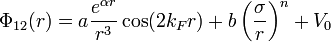$\Phi_{12}(r) = a \frac{e^{\alpha r}}{r^3} \cos (2k_Fr) + b \left( \frac{\sigma}{r} \right)^n + V_0$
 a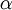$\alpha$$k_F$ b$\sigma$ n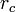$r_c$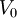$V_0$ Z1 1.58 –0.22 4.120 4.2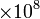$\times 10^8$ 0.331 18.0 2.64909 0.04682632 Z2 1.04 0.33 4.139 4.2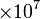$\times 10^7$ 0.348 14.5 2.64488 0.13391543